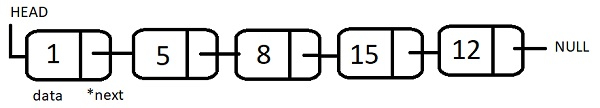# Maximum and Minimum element of a linked list which is divisible by a given number k in C++

A linked list is a linear data structure in which elements are linked via pointers. Each element or node of a linked list has a data part and link, or we can say pointer to the next element in sequence. The elements can take noncontiguous locations in memory.We are given a singly linked list in which there is a data part and link to the next element. The other input is a number K. Task is to find the Maximum and Minimum element of a linked list which is divisible by the number K. The linear linked list can only be traversed in one direction. At each node we will check the divisibility of it’s data part with K. If that number is maximum or minimum found so far then we will update the values of MaxD and MinD.

Input

SList : 5-->2-->10-->12-->3-->20-->7, K=5

Output

Maximum element which is divisible by K : 20
Maximum element which is divisible by K : 5

Explanation − Traversing from the Head node, keep on dividing the data part with K and check if its completely divisible, that is remainder comes 0.

Only 5, 10 and 20 are divisible by 5 out of which 5 is minimum and 20 is maximum.

Input

SList : 12-->2-->5-->18-->3-->144-->7, K=4

Output

Maximum element which is divisible by K : 144
Maximum element which is divisible by K : 12

Explanation − Traversing from the Head node, keep on dividing the data part with K and check if its completely divisible, that is remainder comes 0.

Only 12, and 144 are divisible by 4 out of which 12 is minimum and 144 is maximum.

## Approach used in the below program is as follows

• Create a linked list node. Here we have created a class SLLnode, which has info part and next pointer.

• Create a linked list. Here we have created a class SLList with SLLnode object as it’s member. So SLList consists of SLLnodes.

• Once the SLList is created we call the function Divisible(SLLnode,int) which takes two input parameters head of list and integer K.

• Now inside Divisible we take two variables maxD and minD to store Maximum and Minimum element of a linked list which is divisible by a given number K.

• maxD is initialized with -1 and minD is initialized 9999. This is supposed as range in which input lies.

• Inside for loop we traverse the linked list starting from head. For this variable start is pointing to head.

• Compare the info part of each node with maxD and minD and it’s divisibility with K. If current node’s info is divisible and less than minD update minD with current info part.

• If current node’s info is divisible by K and greater than maxD, update maxD with current info part.

• Print the result obtained in minD and maxD.

## Example

#include<iostream.h>
#include<process.h>
#include<conio.h>
class SLLnode{
public:
int info;
SLLnode *next;
SLLnode(int e1,SLLnode *ptr=0){
info=e1;
next=ptr;
}
};
class SLList{
public:
SLList()
int minD=9999;
int maxD=-1;
for(start;start->next!=NULL;start=start->next){
if ((start->info < minD) && (start->info % K == 0))
minD = start->info;
if ((start->info > maxD) && (start->info % K == 0))
maxD = start->info;
}
cout << "Max Element divisible by K: " << maxD << endl;
cout << "Min Element divisible by K: " << minD;
}
// Driver Code
int main(){
clrscr();
SLList LIST;
int K = 5;
getch();
return 0;
}

## Output

If we run the above code it will generate the following output −

Max Element divisible by K: 90
Min Element divisible by K: 45

Updated on: 14-Aug-2020

90 Views